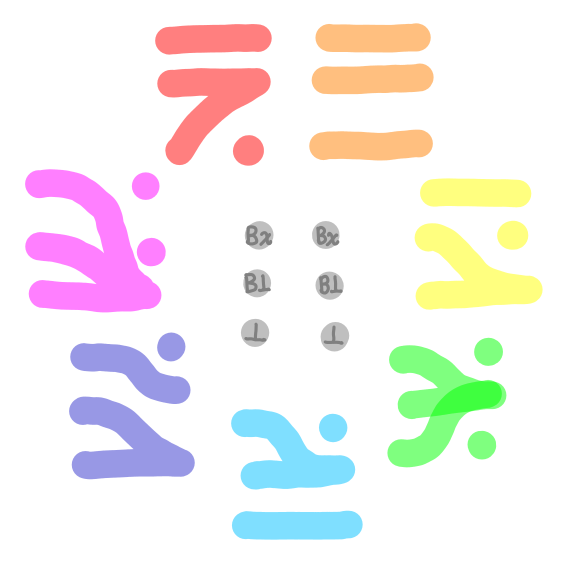the arc of software bends towards understanding

## Joseph and the Amazing Technicolor Box

Consider the following data type in Haskell:

```data Box a = B a
```

How many computable functions of type Box a -> Box a are there? If we strictly use denotational semantics, there are seven:But if we furthermore distinguish the source of the bottom (a very operational notion), some functions with the same denotation have more implementations...

1. Irrefutable pattern match: f ~(B x) = B x. No extras.
2. Identity: f b = b. No extras.
3. Strict: f (B !x) = B x. No extras.
4. Constant boxed bottom: Three possibilities: f _ = B (error "1"); f b = B (case b of B _ -> error "2"); and f b = B (case b of B !x -> error "3").
5. Absent: Two possibilities: f (B _) = B (error "4"); and f (B x) = B (x `seq` error "5").
6. Strict constant boxed bottom: f (B !x) = B (error "6").
7. Bottom: Three possibilities: f _ = error "7"; f (B _) = error "8"; and f (B !x) = error "9".

List was ordered by colors of the rainbow. If this was hieroglyphics to you, may I interest you in this blog post?

Postscript. GHC can and will optimize f b = B (case b of B !x -> error "3"), f (B x) = B (x `seq` error "5") and f (B !x) = error "9" into alternative forms, because in general we don't say if seq (error "1") (error "2") is semantically equivalent error "1" or error "2": any one is possible due to imprecise exceptions. But if you really care, you can use pseq. However, even with exception set semantics, there are more functions in this "refined" view of the normal denotational semantics.